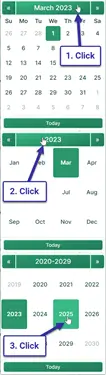# Accurate Compound Interest Calculator

Savings, Investment or Loan Interest Calculations

This compound interest calculator calculates interest between any two dates. A dozen compounding periods are supported (did we miss any? :). You can also enter negative interest rates.

Because this calculator is date sensitive, and because it supports many compounding periods, it is a suitable tool for calculating the compound interest owed on a debt. You can use it to calculate accrued interest from a point in time when the balance is known. Because this calculator allows for odd days (example three months plus five days), you may calculate interest due for any investment or debt. More details below the calculator

© 2022, Pine Grove Software, LLC
\$ : mm/dd/yyyy

Original Size

## Compound Interest

Compound interest means that interest gets paid (or is earned) on previously unpaid interest.To Quickly
Pick a Date

For example, if the interest rate is 2% and you start with \$1,000 after the end of a year, you'll earn or owe \$20 in interest (using annual compounding). Then at the end of two years, assuming there have been no withdrawals (or payments) you earn \$20.40, not \$20. The previous period's interest earned interest as well.

This pattern is called compounding, and it repeats as long as the money stays invested, or the debtor owes on the debt.

If you are an investor, you want to compound interest. If you are a debtor, you want to avoid it, particularly if you ever miss a payment or a payment is not enough to cover the interest due.

You can use this online interest calculator as a:

• apy calculator
• daily interest calculator
• investment interest calculator
• loan interest calculator
• negative interest rate calculator
• savings account interest calculator

As a side benefit to this calculator's date accuracy, you can use it for date math calculations. That is, given two dates, it will calculate the number of days between them, or it will find the date that is "X" days from the first date.

## 61 Comments on “Compound Interest Calculator”

Join the conversation. Tell me what you think.